In :
%matplotlib inline
from preamble import *


## 2. Supervised Learning¶

### 2.2 Generalization, Overfitting and Underfitting¶### 2.3 Supervised Machine Learning Algorithms¶

#### 2.3.1 Some Sample Datasets¶

• forge 데이터셋
In :
# generate dataset
X, y = mglearn.datasets.make_forge()
print("X.shape: {}".format(X.shape))
print("X:\n{}".format(X))
print("y.shape: {}".format(y.shape))
print("y:\n{}".format(y))

# plot dataset
plt.figure(figsize=(10, 5))
mglearn.discrete_scatter(X[:, 0], X[:, 1], y)
plt.legend(["Class 0", "Class 1"], loc=4)
plt.xlabel("First feature")
plt.ylabel("Second feature")
plt.show()

X.shape: (26, 2)
X:
[[ 9.963  4.597]
[11.033 -0.168]
[11.542  5.211]
[ 8.693  1.543]
[ 8.106  4.287]
[ 8.31   4.806]
[11.93   4.649]
[ 9.673 -0.203]
[ 8.348  5.134]
[ 8.675  4.476]
[ 9.177  5.093]
[10.24   2.455]
[ 8.689  1.487]
[ 8.922 -0.64 ]
[ 9.491  4.332]
[ 9.257  5.133]
[ 7.998  4.853]
[ 8.184  1.296]
[ 8.734  2.492]
[ 9.323  5.098]
[10.064  0.991]
[ 9.5   -0.264]
[ 8.345  1.638]
[ 9.502  1.938]
[ 9.151  5.498]
[11.564  1.339]]
y.shape: (26,)
y:
[1 0 1 0 0 1 1 0 1 1 1 1 0 0 1 1 1 0 0 1 0 0 0 0 1 0]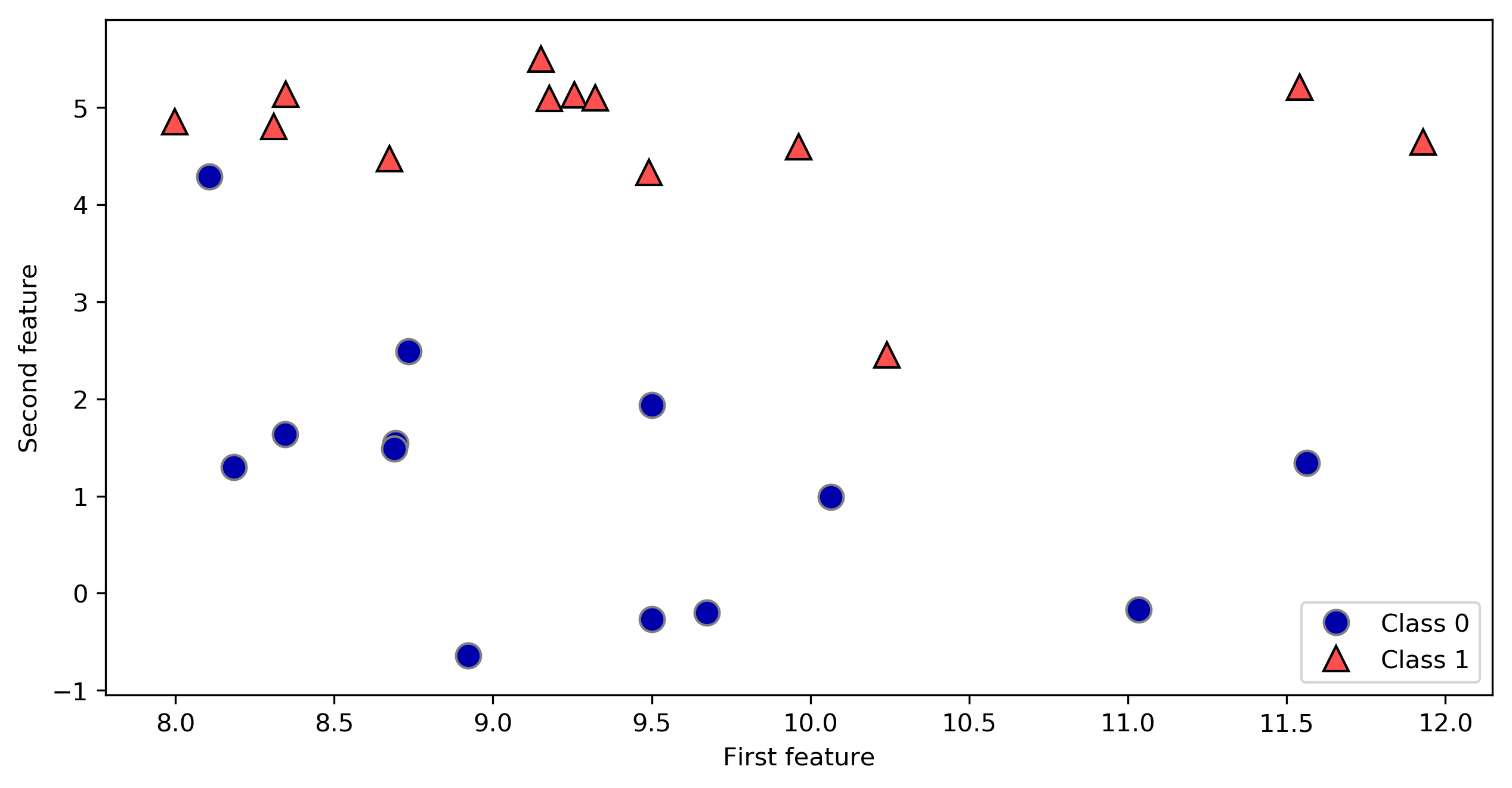• wave 데이터셋
In :
X, y = mglearn.datasets.make_wave(n_samples=40)
print("X.shape: {}".format(X.shape))
print("X:\n{}".format(X))
print("y.shape: {}".format(y.shape))
print("y:\n{}".format(y))

plt.figure(figsize=(10, 5))
plt.plot(X, y, 'o')
plt.ylim(-3, 3)
plt.xlabel("Feature")
plt.ylabel("Target")
plt.show()

X.shape: (40, 1)
X:
[[-0.753]
[ 2.704]
[ 1.392]
[ 0.592]
[-2.064]
[-2.064]
[-2.651]
[ 2.197]
[ 0.607]
[ 1.248]
[-2.876]
[ 2.819]
[ 1.995]
[-1.726]
[-1.909]
[-1.9  ]
[-1.175]
[ 0.149]
[-0.408]
[-1.253]
[ 0.671]
[-2.163]
[-1.247]
[-0.802]
[-0.264]
[ 1.711]
[-1.802]
[ 0.085]
[ 0.554]
[-2.721]
[ 0.645]
[-1.977]
[-2.61 ]
[ 2.693]
[ 2.794]
[ 1.85 ]
[-1.172]
[-2.414]
[ 1.105]
[-0.359]]
y.shape: (40,)
y:
[-0.448  0.331  0.779  0.035 -1.388 -2.472 -1.527  1.494  1.     0.23
-1.06   0.779  0.754 -1.514 -1.673 -0.905  0.084 -0.527 -0.541 -0.341
0.218 -1.125  0.373  0.098 -0.986  0.967 -1.135  0.698  0.437 -0.957
0.035 -2.086 -0.474  1.537  0.869  1.877  0.095 -1.415  0.254  0.094]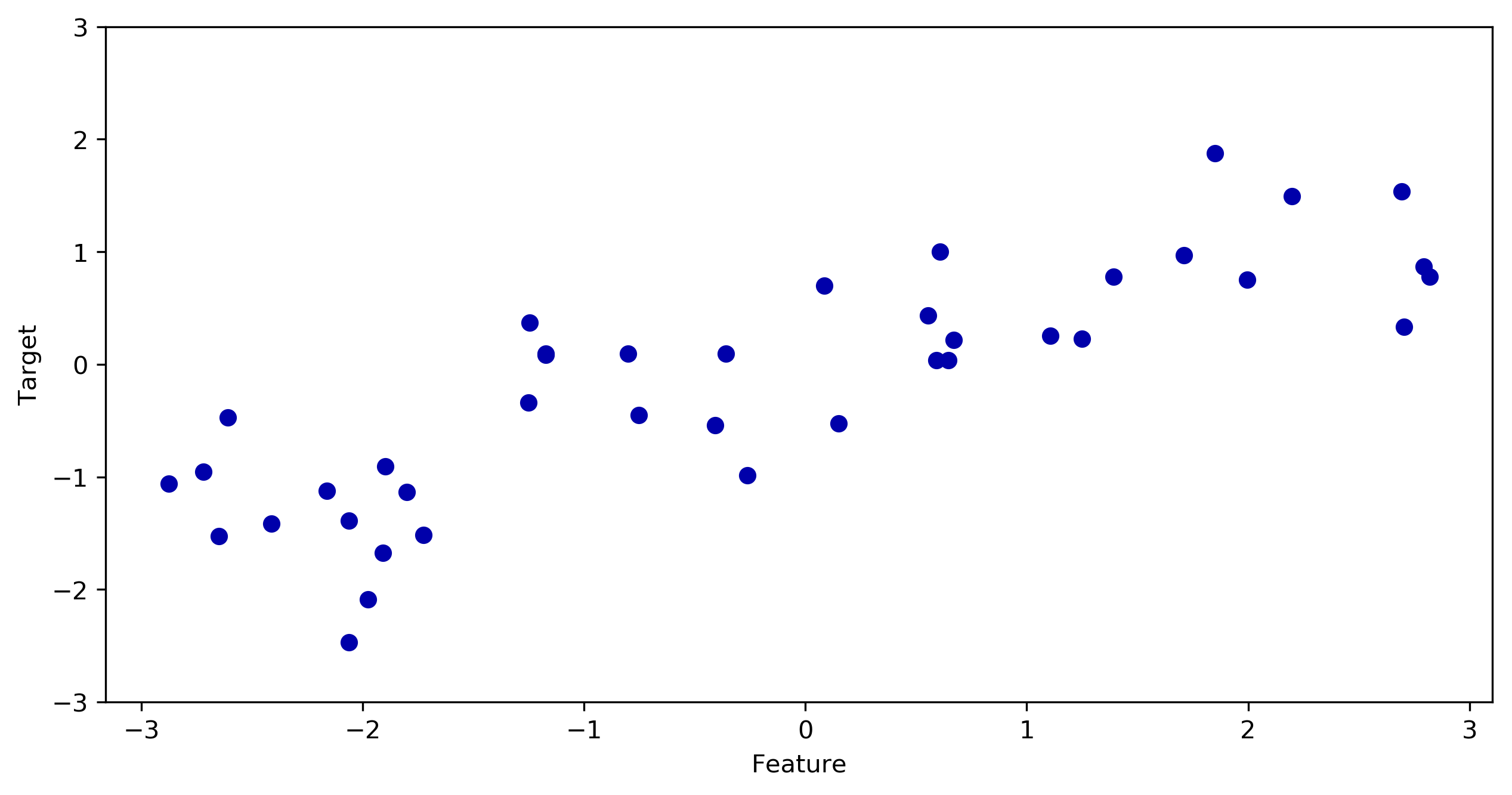• Wisconsin Breast Cancer 데이터셋
In :
from sklearn.datasets import load_breast_cancer
print("cancer.keys(): {}".format(cancer.keys()))

cancer.keys(): dict_keys(['data', 'target', 'target_names', 'DESCR', 'feature_names'])

In :
print("Shape of cancer data: {}".format(cancer.data.shape))

Shape of cancer data: (569, 30)

In :
print("Sample counts per class:\n{}".format(
{n: v for n, v in zip(cancer.target_names, np.bincount(cancer.target))}
))

Sample counts per class:
{'malignant': 212, 'benign': 357}

In :
print("Feature names:\n{}".format(cancer.feature_names))

Feature names:
['mean radius' 'mean texture' 'mean perimeter' 'mean area'
'mean smoothness' 'mean compactness' 'mean concavity'
'mean concave points' 'mean symmetry' 'mean fractal dimension'
'radius error' 'texture error' 'perimeter error' 'area error'
'smoothness error' 'compactness error' 'concavity error'
'concave points error' 'symmetry error' 'fractal dimension error'
'worst radius' 'worst texture' 'worst perimeter' 'worst area'
'worst smoothness' 'worst compactness' 'worst concavity'
'worst concave points' 'worst symmetry' 'worst fractal dimension']

• Boston Housing 데이터셋
In :
from sklearn.datasets import load_boston
print("boston.keys(): {}".format(boston.keys()))

boston.keys(): dict_keys(['data', 'target', 'feature_names', 'DESCR'])

In :
print("Feature Names: {}".format(boston.feature_names))
print("Feature Names: {}".format(boston.DESCR))

Feature Names: ['CRIM' 'ZN' 'INDUS' 'CHAS' 'NOX' 'RM' 'AGE' 'DIS' 'RAD' 'TAX' 'PTRATIO'
'B' 'LSTAT']
Feature Names: Boston House Prices dataset
===========================

Notes
------
Data Set Characteristics:

:Number of Instances: 506

:Number of Attributes: 13 numeric/categorical predictive

:Median Value (attribute 14) is usually the target

:Attribute Information (in order):
- CRIM     per capita crime rate by town
- ZN       proportion of residential land zoned for lots over 25,000 sq.ft.
- INDUS    proportion of non-retail business acres per town
- CHAS     Charles River dummy variable (= 1 if tract bounds river; 0 otherwise)
- NOX      nitric oxides concentration (parts per 10 million)
- RM       average number of rooms per dwelling
- AGE      proportion of owner-occupied units built prior to 1940
- DIS      weighted distances to five Boston employment centres
- TAX      full-value property-tax rate per $10,000 - PTRATIO pupil-teacher ratio by town - B 1000(Bk - 0.63)^2 where Bk is the proportion of blacks by town - LSTAT % lower status of the population - MEDV Median value of owner-occupied homes in$1000's

:Missing Attribute Values: None

:Creator: Harrison, D. and Rubinfeld, D.L.

This is a copy of UCI ML housing dataset.
http://archive.ics.uci.edu/ml/datasets/Housing

This dataset was taken from the StatLib library which is maintained at Carnegie Mellon University.

The Boston house-price data of Harrison, D. and Rubinfeld, D.L. 'Hedonic
prices and the demand for clean air', J. Environ. Economics & Management,
vol.5, 81-102, 1978.   Used in Belsley, Kuh & Welsch, 'Regression diagnostics
...', Wiley, 1980.   N.B. Various transformations are used in the table on
pages 244-261 of the latter.

The Boston house-price data has been used in many machine learning papers that address regression
problems.

**References**

- Belsley, Kuh & Welsch, 'Regression diagnostics: Identifying Influential Data and Sources of Collinearity', Wiley, 1980. 244-261.
- Quinlan,R. (1993). Combining Instance-Based and Model-Based Learning. In Proceedings on the Tenth International Conference of Machine Learning, 236-243, University of Massachusetts, Amherst. Morgan Kaufmann.
- many more! (see http://archive.ics.uci.edu/ml/datasets/Housing)


In :
print("Data shape: {}".format(boston.data.shape))
print("Target shape: {}".format(boston.target.shape))

Data shape: (506, 13)
Target shape: (506,)

In :
X, y = mglearn.datasets.load_extended_boston()
print("X.shape: {}".format(X.shape))

X.shape: (506, 104)


### 2.3.2 k-Nearest Neighbor¶

#### k-Neighbors Classification¶

In :
plt.figure(figsize=(10, 5))
mglearn.plots.plot_knn_classification(n_neighbors=1)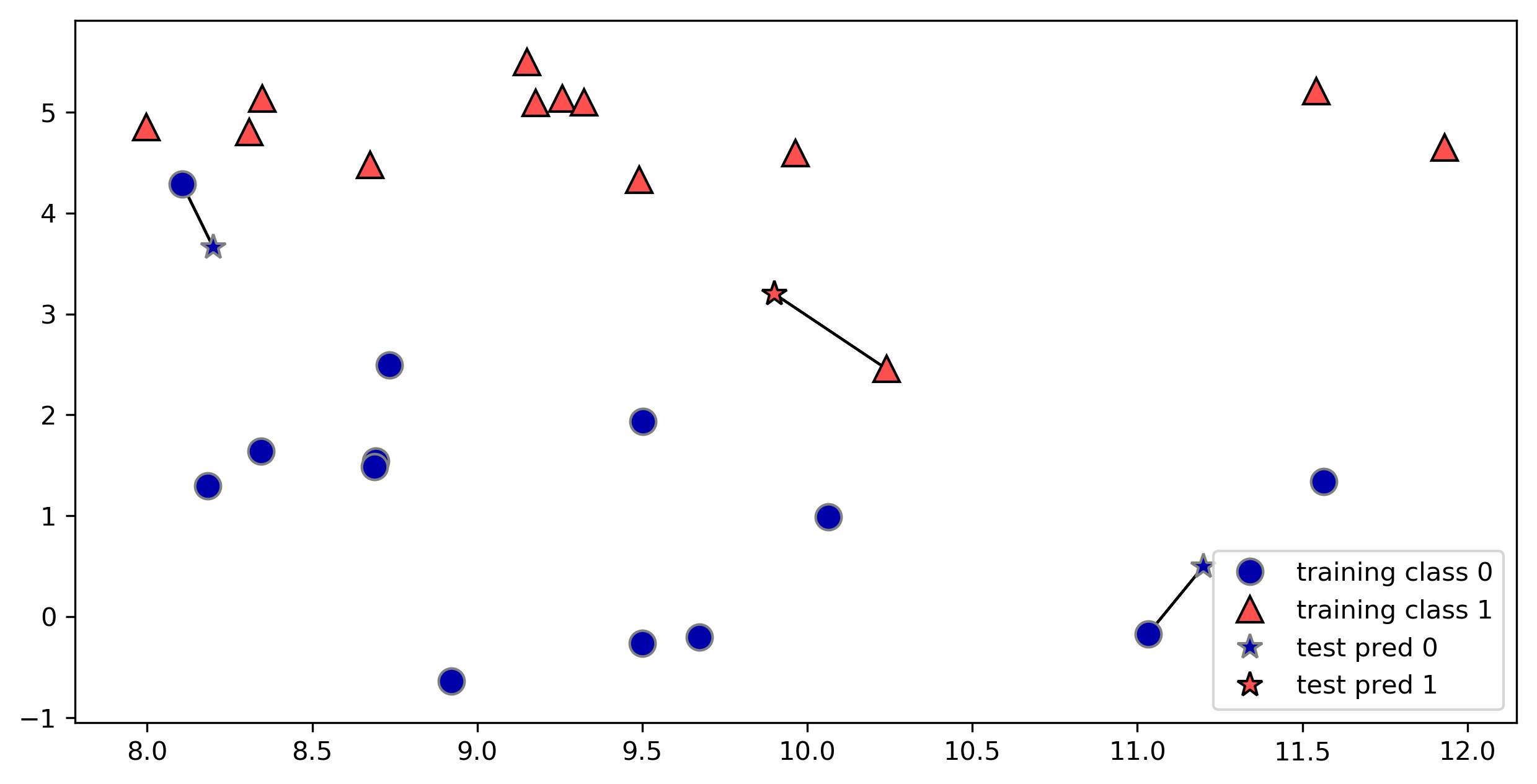In :
plt.figure(figsize=(10, 5))
mglearn.plots.plot_knn_classification(n_neighbors=3)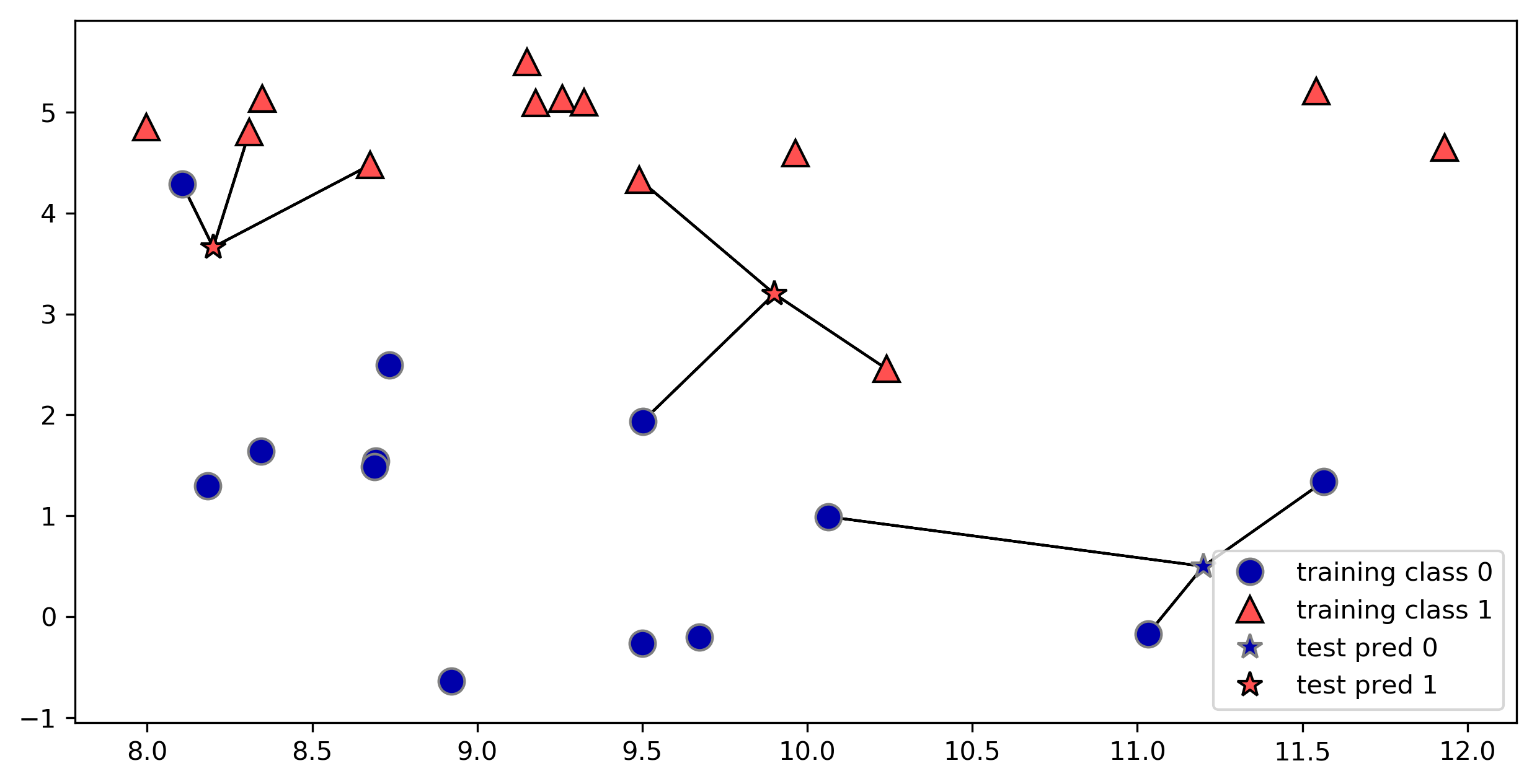In :
from sklearn.model_selection import train_test_split
# X --> (26, 2)
# Y --> (26,)
X, y = mglearn.datasets.make_forge()

X_train, X_test, y_train, y_test = train_test_split(X, y, random_state=0)
print("X_train shape: {}".format(X_train.shape))
print("y_train shape: {}".format(y_train.shape))

print()

print("X_test shape: {}".format(X_test.shape))
print("y_test shape: {}".format(y_test.shape))

X_train shape: (19, 2)
y_train shape: (19,)

X_test shape: (7, 2)
y_test shape: (7,)

In :
from sklearn.neighbors import KNeighborsClassifier
clf = KNeighborsClassifier(n_neighbors=3)

In :
clf.fit(X_train, y_train)

Out:
KNeighborsClassifier(algorithm='auto', leaf_size=30, metric='minkowski',
metric_params=None, n_jobs=1, n_neighbors=3, p=2,
weights='uniform')
In :
print("Test set predictions: {}".format(clf.predict(X_test)))

Test set predictions: [1 0 1 0 1 0 0]

In :
print("Test set accuracy: {:.2f}".format(clf.score(X_test, y_test)))

Test set accuracy: 0.86

• clf.score() outputs 'R²' score
• 1.0 : Perfect Prediction
• 0.0 : Prediction to the mean of the given y values
• $R^2 = 1 - {\frac{RSS}{TSS}}$
• RSS is the residual sum of squares $∑(y - f(x))²$
• TSS is the total sum of squares $∑(y - mean(y))²$.

#### Analyzing KNeighborsClassifier¶

In :
fig, axes = plt.subplots(1, 3, figsize=(10, 3))

for n_neighbors, ax in zip([1, 3, 9], axes):
# the fit method returns the object self, so we can instantiate
# and fit in one line:
clf = KNeighborsClassifier(n_neighbors=n_neighbors).fit(X, y)
mglearn.plots.plot_2d_separator(clf, X, fill=True, eps=0.5, ax=ax, alpha=.4)
mglearn.discrete_scatter(X[:, 0], X[:, 1], y, ax=ax)
ax.set_title("{} neighbor(s)".format(n_neighbors))
ax.set_xlabel("feature 0")
ax.set_ylabel("feature 1")
axes.legend(loc=3)
plt.show()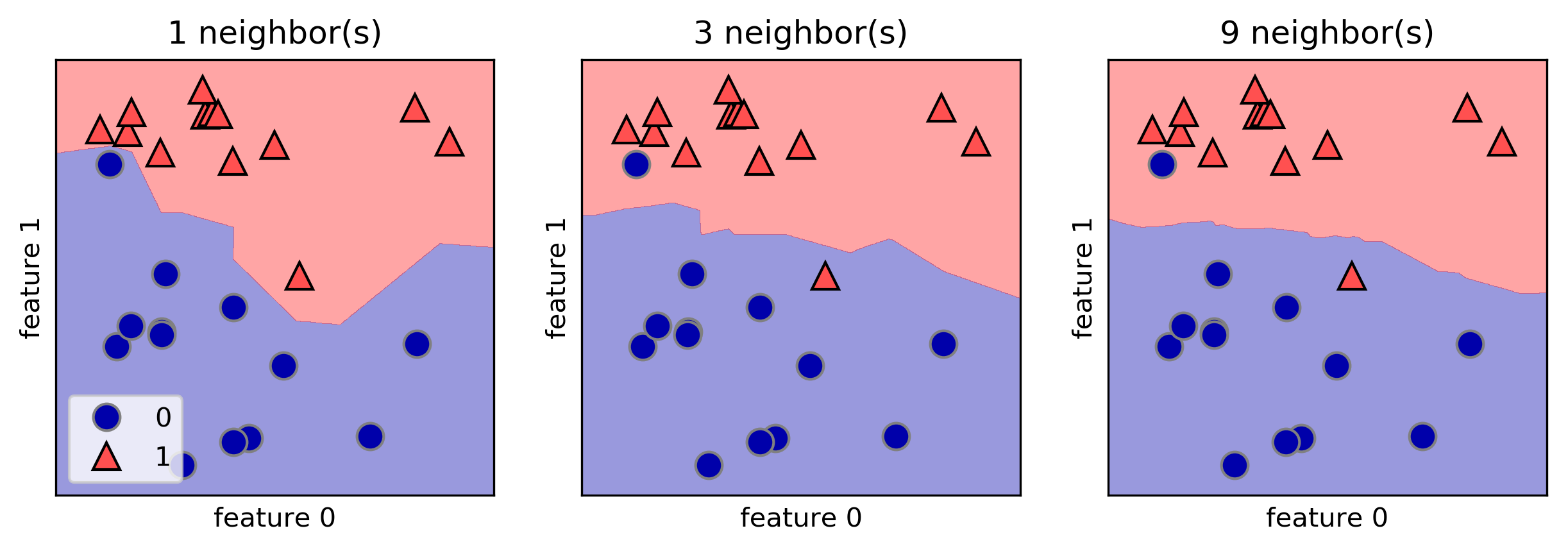In :
from sklearn.datasets import load_breast_cancer

X_train, X_test, y_train, y_test = train_test_split(
cancer.data,
cancer.target,
stratify=cancer.target,
random_state=66
)

training_accuracy = []
test_accuracy = []
# try n_neighbors from 1 to 10
neighbors_settings = range(1, 11)

for n_neighbors in neighbors_settings:
# build the model
clf = KNeighborsClassifier(n_neighbors=n_neighbors)
clf.fit(X_train, y_train)
# record training set accuracy
training_accuracy.append(clf.score(X_train, y_train))
# record generalization accuracy
test_accuracy.append(clf.score(X_test, y_test))

plt.figure(figsize=(8, 6), dpi=80, facecolor='w', edgecolor='k')
plt.plot(neighbors_settings, training_accuracy, label="training accuracy")
plt.plot(neighbors_settings, test_accuracy, label="test accuracy")
plt.ylabel("Accuracy")
plt.xlabel("n_neighbors")
plt.legend()
plt.show()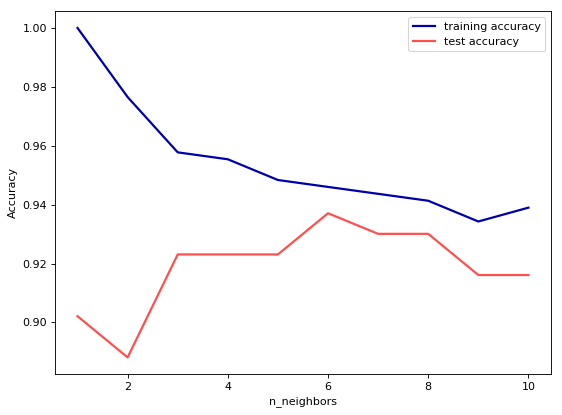#### k-Neighbors Regression¶

In :
mglearn.plots.plot_knn_regression(n_neighbors=1)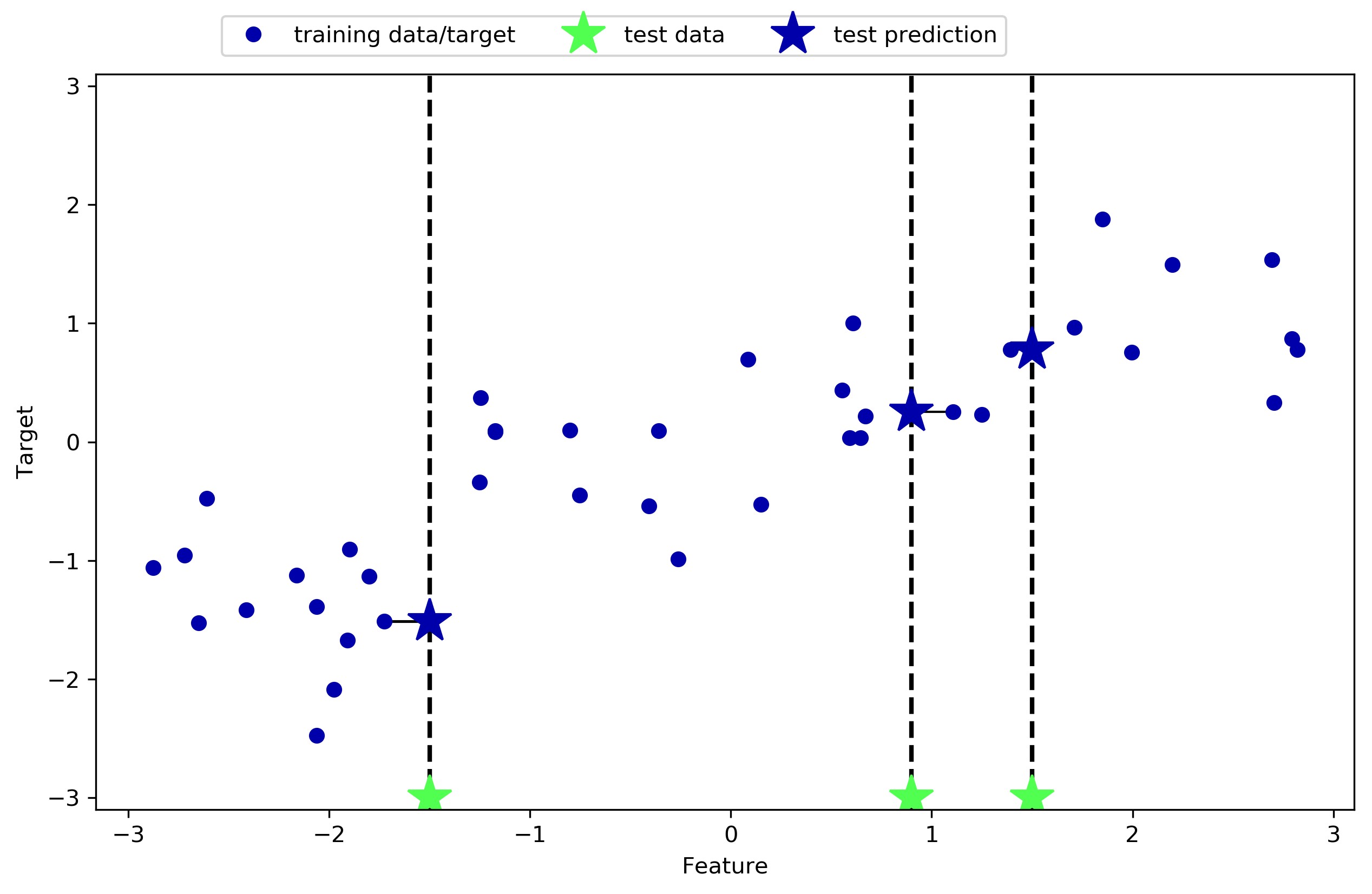In :
mglearn.plots.plot_knn_regression(n_neighbors=3)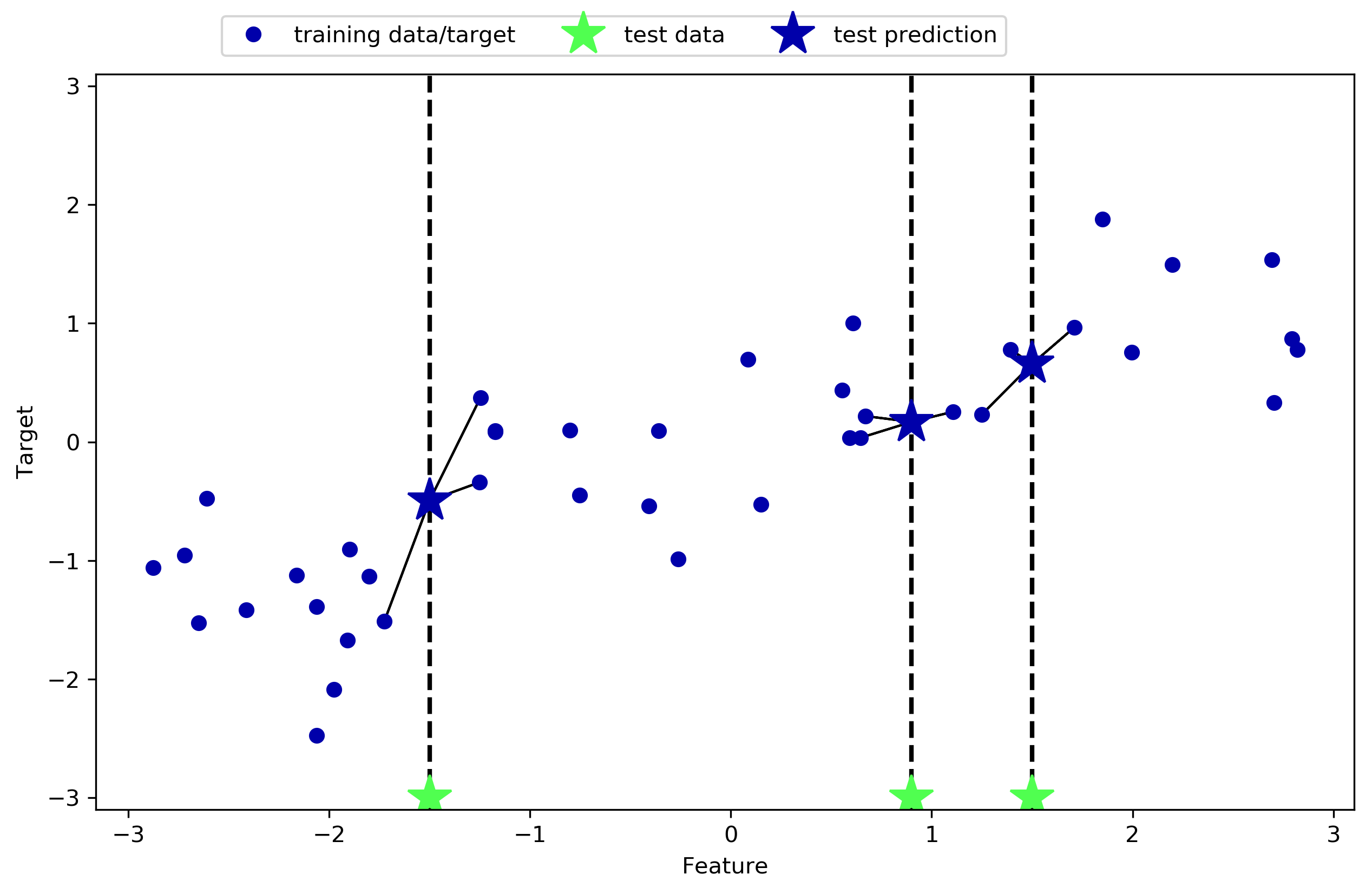In :
from sklearn.neighbors import KNeighborsRegressor

X, y = mglearn.datasets.make_wave(n_samples=40)

# split the wave dataset into a training and a test set
X_train, X_test, y_train, y_test = train_test_split(X, y, random_state=0)

# instantiate the model and set the number of neighbors to consider to 3:
reg = KNeighborsRegressor(n_neighbors=3)
# fit the model using the training data and training targets:
reg.fit(X_train, y_train)

Out:
KNeighborsRegressor(algorithm='auto', leaf_size=30, metric='minkowski',
metric_params=None, n_jobs=1, n_neighbors=3, p=2,
weights='uniform')
In :
print("Test set predictions:\n{}".format(reg.predict(X_test)))

Test set predictions:
[-0.054  0.357  1.137 -1.894 -1.139 -1.631  0.357  0.912 -0.447 -1.139]

In :
print("Test set R^2: {:.2f}".format(reg.score(X_test, y_test)))

Test set R^2: 0.83


#### Analyzing KNeighborsRegressor¶

In :
fig, axes = plt.subplots(1, 3, figsize=(15, 4))
# create 1,000 data points, evenly spaced between -3 and 3
line = np.linspace(-3, 3, 1000).reshape(-1, 1)
for n_neighbors, ax in zip([1, 3, 9], axes):
# make predictions using 1, 3, or 9 neighbors
reg = KNeighborsRegressor(n_neighbors=n_neighbors)
reg.fit(X_train, y_train)
ax.plot(line, reg.predict(line))
ax.plot(X_train, y_train, '^', c=mglearn.cm2(0), markersize=8)
ax.plot(X_test, y_test, 'v', c=mglearn.cm2(1), markersize=8)

ax.set_title(
"{} neighbor(s)\n train score: {:.2f} test score: {:.2f}".format(
n_neighbors, reg.score(X_train, y_train),
reg.score(X_test, y_test)))
ax.set_xlabel("Feature")
ax.set_ylabel("Target")
axes.legend(["Model predictions", "Training data/target",
"Test data/target"], loc="best")

Out:
<matplotlib.legend.Legend at 0x11bd61390>